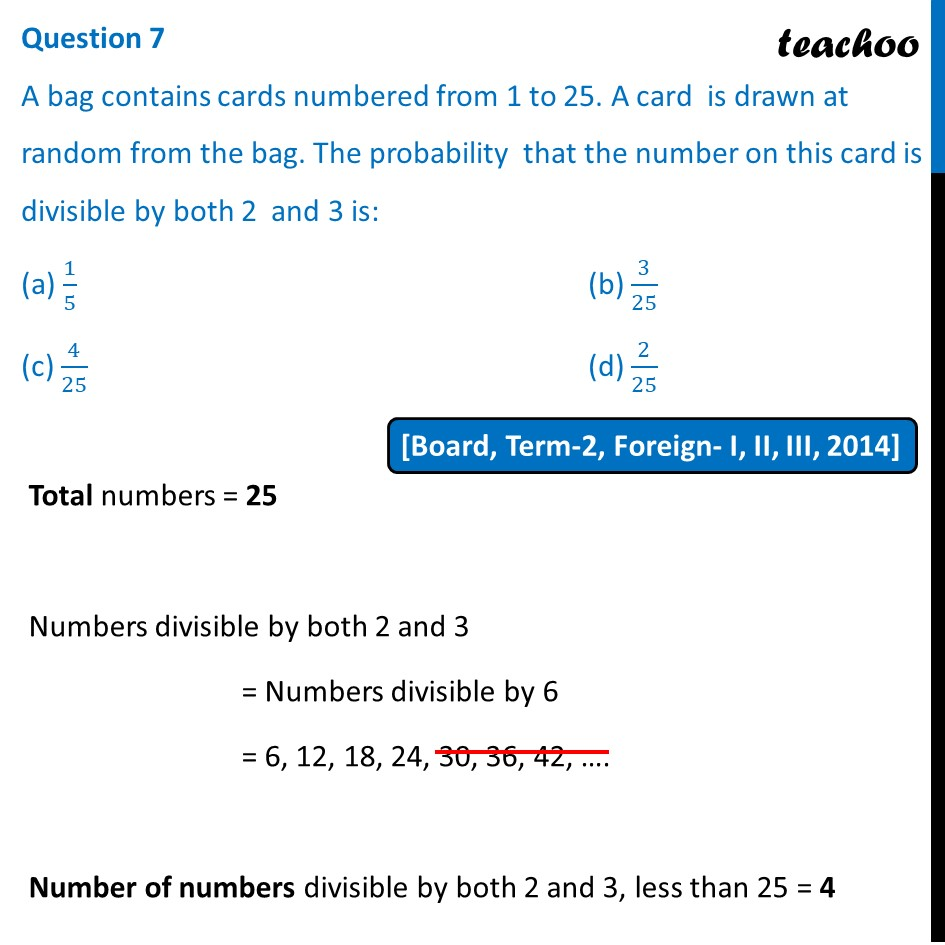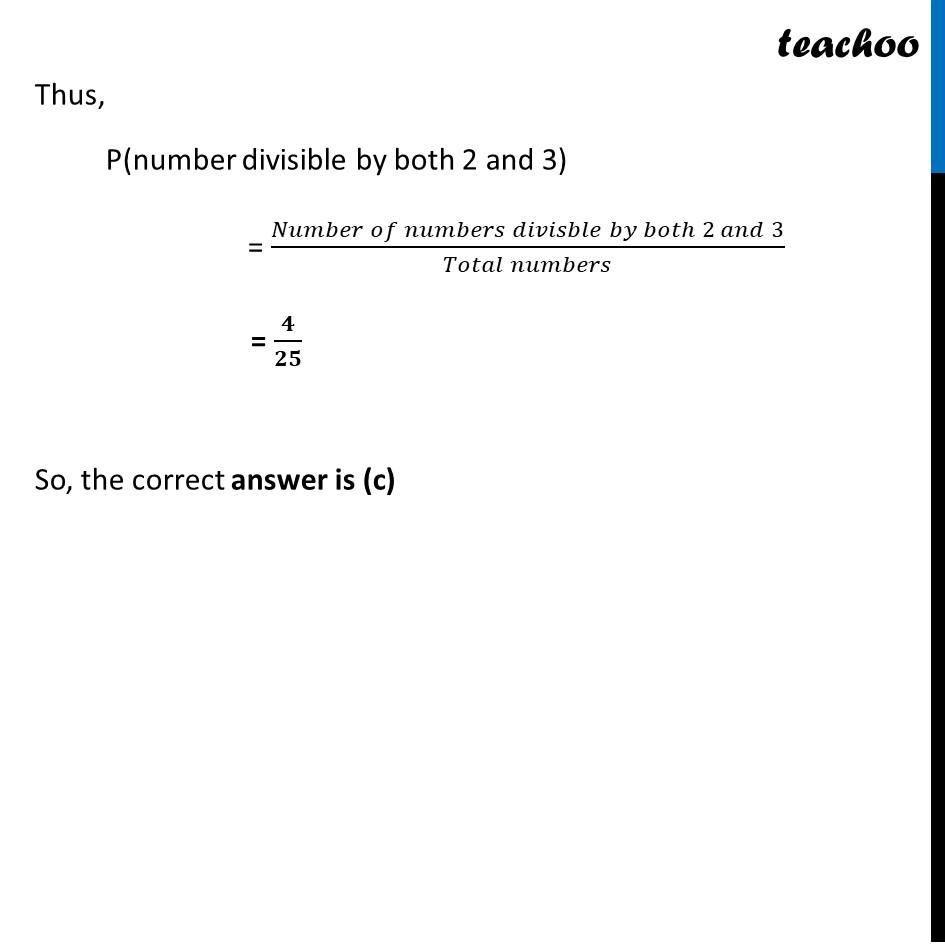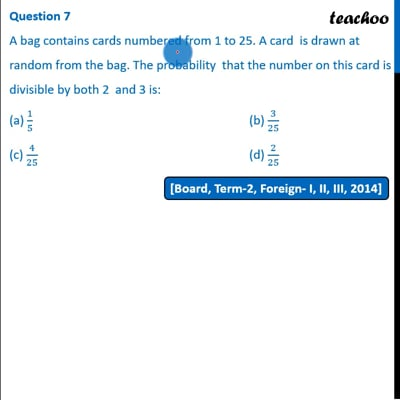Past Year MCQ

Chapter 15 Class 10 Probability
Serial order wise

## (c) 4/25                                                  (d) 2/25This video is only available for Teachoo black users

Maths Crash Course - Live lectures + all videos + Real time Doubt solving!

### Transcript

Question 7 A bag contains cards numbered from 1 to 25. A card is drawn at random from the bag. The probability that the number on this card is divisible by both 2 and 3 is: (a) 1/5 (b) 3/25 (c) 4/25 (d) 2/25Total numbers = 25 Numbers divisible by both 2 and 3 = Numbers divisible by 6 = 6, 12, 18, 24, 30, 36, 42, …. Number of numbers divisible by both 2 and 3, less than 25 = 4 Thus, P(number divisible by both 2 and 3) = (𝑁𝑢𝑚𝑏𝑒𝑟 𝑜𝑓 𝑛𝑢𝑚𝑏𝑒𝑟𝑠 𝑑𝑖𝑣𝑖𝑠𝑏𝑙𝑒 𝑏𝑦 𝑏𝑜𝑡ℎ 2 𝑎𝑛𝑑 3)/(𝑇𝑜𝑡𝑎𝑙 𝑛𝑢𝑚𝑏𝑒𝑟𝑠) = 𝟒/𝟐𝟓 So, the correct answer is (c)